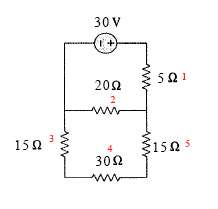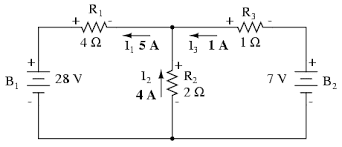# Combined Circuit Problem

## Homework Statement## Homework Equations

IR=V
series circuit
Vt = V1 + V2 + V3...
It = I1 = I2 = I3...
Rt = R1 + R2 + R3...

parallel circuit
Vt = V1 = V2 = V3...
It = I1 + I2 + I3...
Rt = 1/R1 + 1/R2 + 1/R3...

## The Attempt at a Solution

i honestly have no idea where to start. may someone pls help me?

phyzguy
Can you identify any resistors which are in series and can be combined?

is 2 and 4 in a parallel circuit as well as 3 and 5?

Here is something which helped me a lot when I was solving circuits
"Analyse how he current flows.If you find the same current going into different resistors they are in series,If different current flow flow through them they are in parallel"

Here is something which helped me a lot when I was solving circuits
"Analyse how he current flows.If you find the same current going into different resistors they are in series,If different current flow flow through them they are in parallel"
i'm not sure how to tell that apart.

phyzguy
i'm not sure how to tell that apart.
When the current reaches the bottom end of resistor 5, where will it go?

When the current reaches the bottom end of resistor 5, where will it go?
back to resistor 1?

phyzguy
back to resistor 1?

No. I mean the bottom end of 5, in the lower right corner. The current flows down through 5, and then where?

No. I mean the bottom end of 5, in the lower right corner. The current flows down through 5, and then where?
oh to resistor 4?

phyzguy
oh to resistor 4?

Right. And then when it leaves 4, where does it go?

resistor 3 and when it leaves 3, it goes to 2? and when it leaves 2, it goes to 1?

phyzguy
resistor 3 and when it leaves 3, it goes to 2? and when it leaves 2, it goes to 1?

Not quite. When it leaves 3, there are two places it can go. But, looking at sidt36's post #4, what can you say about 3, 4, and 5?

3, 4, and 5 are parallel to each other. does that mean that 1 and 2 are series?

phyzguy
3, 4, and 5 are parallel to each other

does that mean that 1 and 2 are series?

No.

No!

No.
is it the opposite?

phyzguy
I'm not going to do the problem for you.

I'm not going to do the problem for you.
yes, i understand that but i don't get what makes a circuit a series or parallel.Try making a diagram like this and check where the the current is going

And I hope you dont mind if I ask which grade you are in because this should be pretty trivial for 10th grade student

And I hope you dont mind if I ask which grade you are in because this should be pretty trivial for 10th grade student
i am in 10th grade and i recently just learned this

gneill
Mentor

## Homework Statement:
:

## The Attempt at a Solution

i honestly have no idea where to start. may someone pls help me?
You haven't stated what you are trying to solve for. What do you need to find? What is the actual problem statement?

Well keep practicing

I recommend a video